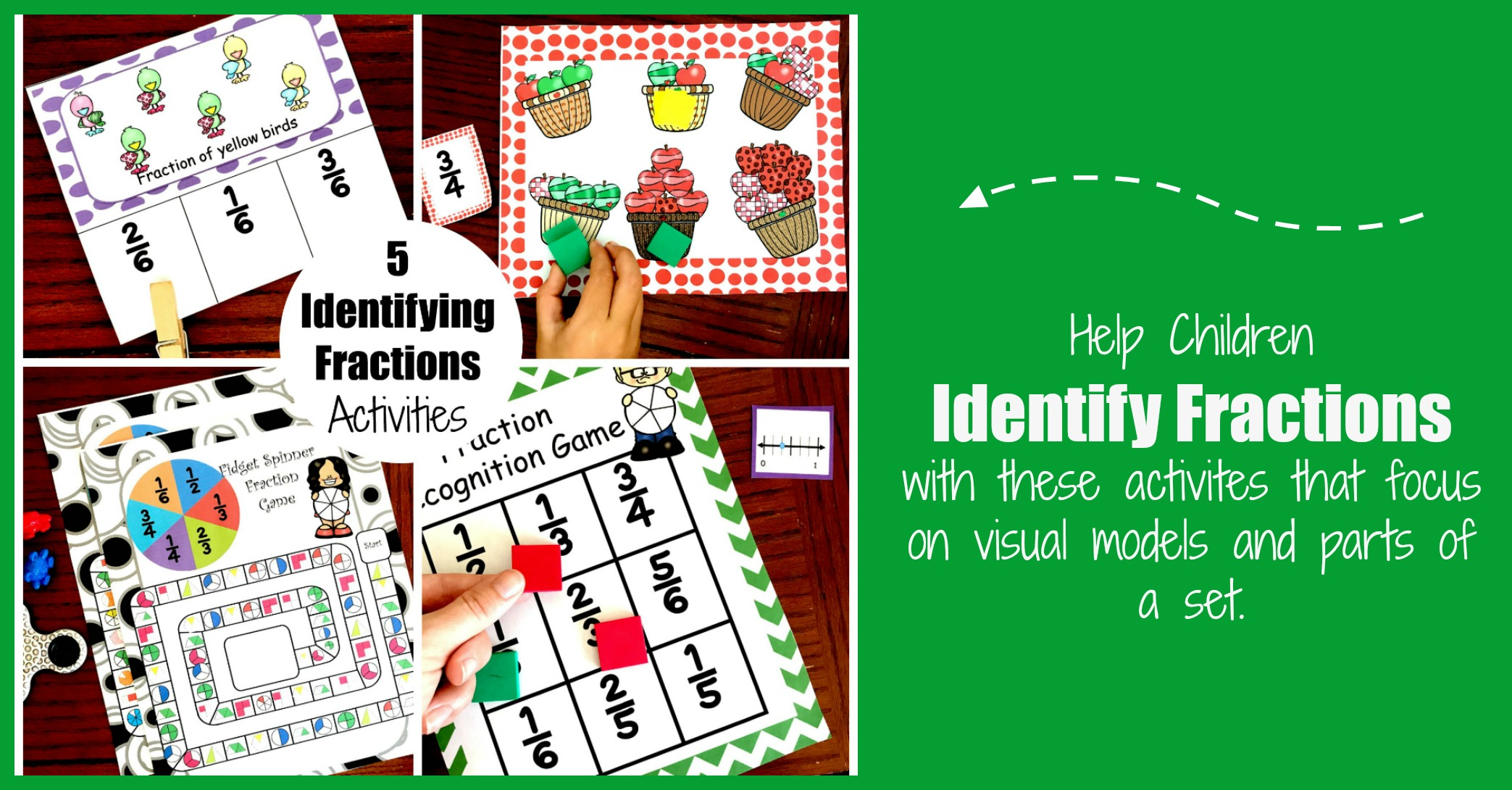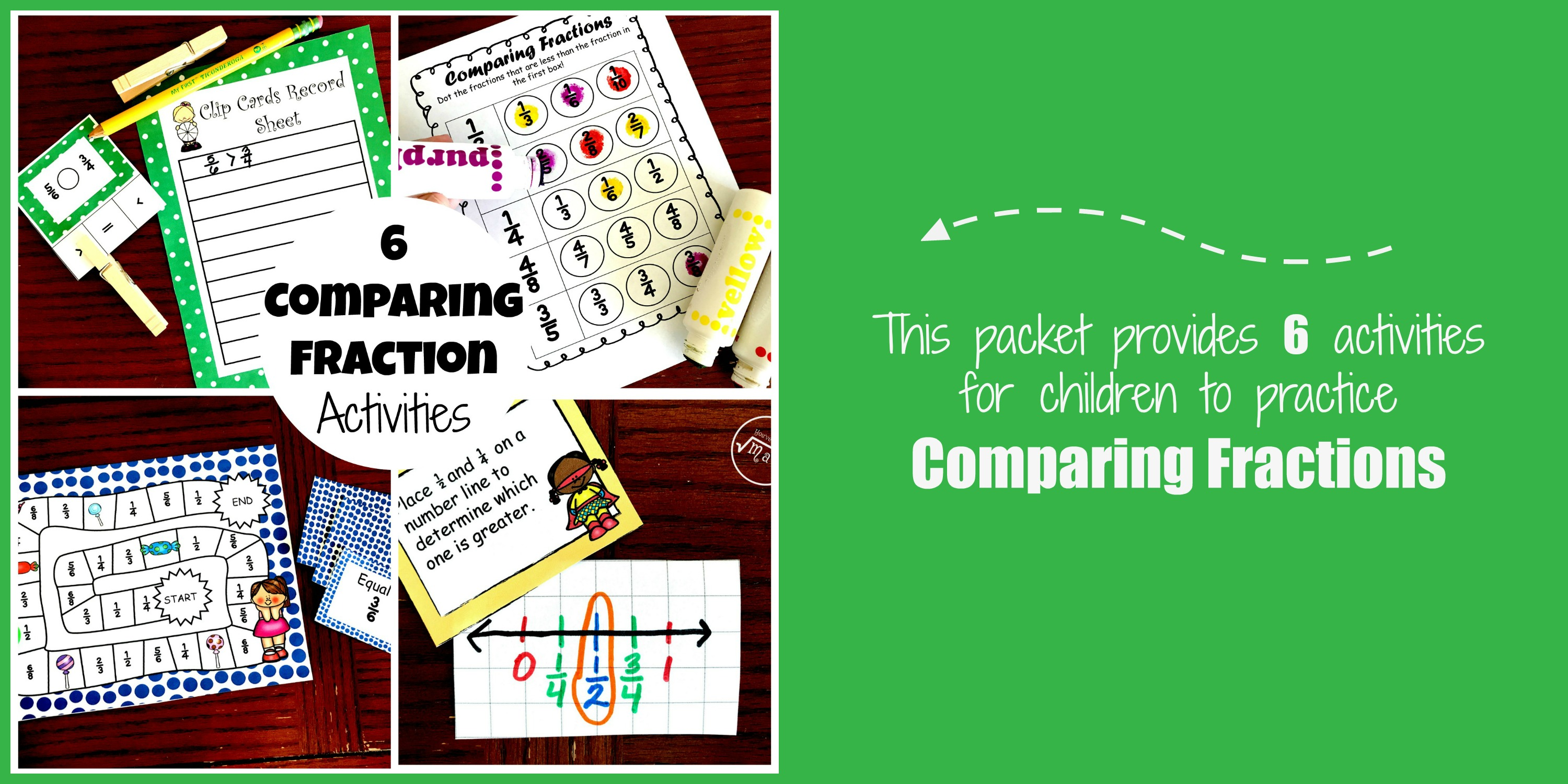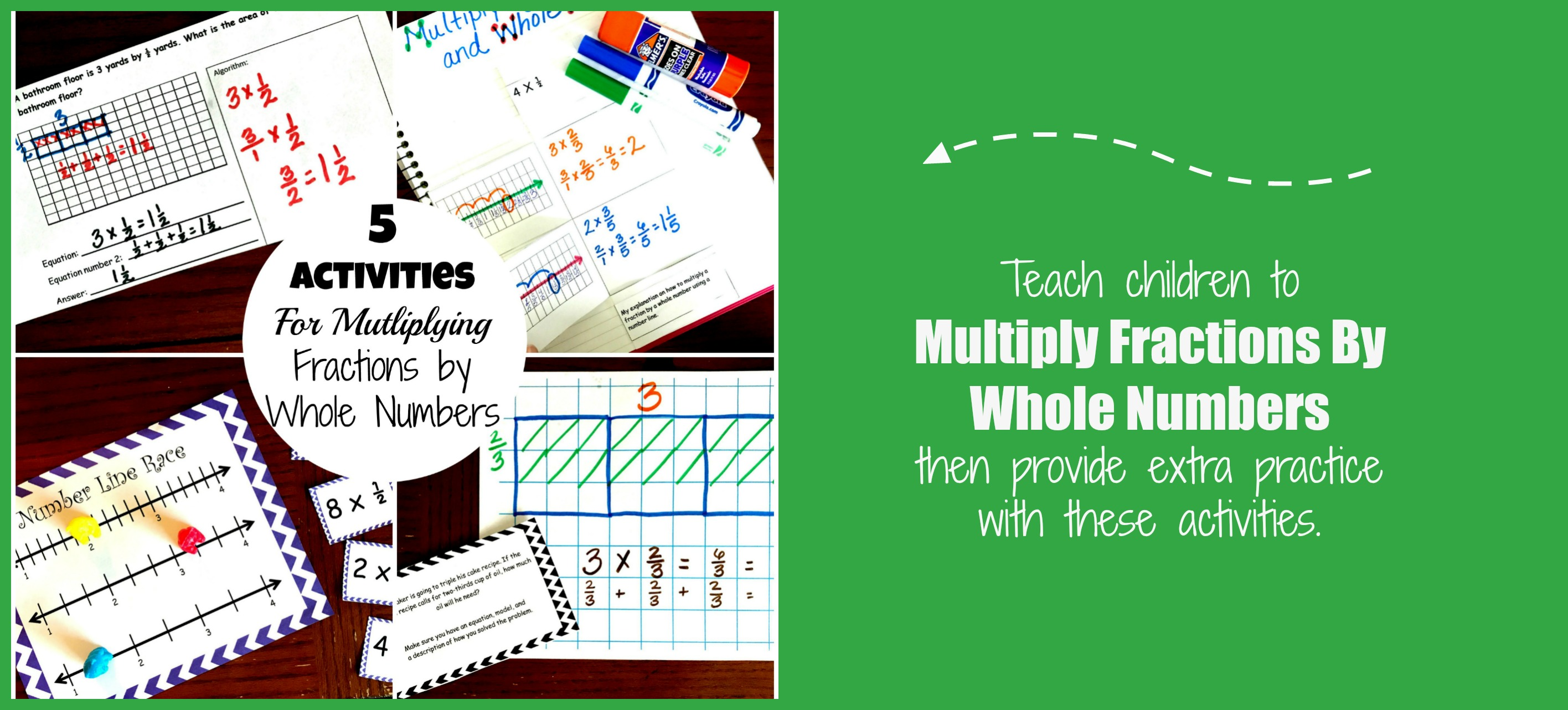Home » Math » 100 Fraction Activities To Help Children Master Fractions

100 Fraction Activities To Help Children Master Fractions

It is important to build fraction sense, and these 100 fraction activities will help your students add, subtract, multiply, divide fractions and much more.

Introducing FractionsThere are numerous fraction activities to help teachers and parents introduce fractions…and they are fun too. There are STEM projects, art projects, and of course games. No matter how you choose to expose your little ones to the world of fractions, here are some concepts you may want to cover.

1. First, children need to understand that a fraction is EQUAL parts of a whole.
2. Next, make sure children understand what numerators and denominators represent.
3. Finally, make sure your activities our hands on and allow children to see fractions in numerous situations. Using visual models, number lines, parts of a set, and arrays are wonderful ways to show children that fractions can be used in situations other than pizzas.

Books are a wonderful way to introduce fractions, and there are so many fraction activities that can be done based on books.

I love this activity based on Twinderella and this one based on Full House. So when you start planning to introduce fractions, grab a few books and enjoy reading with your kiddos.

These are a few we love.

Fidget Spinner Visual Model Game by You’ve Got This Math

Heart Fractions From Life Over C’s

Pizza Fraction Clip Cards from Playdough to Plato

Chocolate Fractions Pack by Life Over C’s

Fraction BINGO by School Time Snippets

Roll and Fill Fraction Game by School Time Snippets

Math Measuring Story With Fraction Work by Wise Owl Factory

Leomande Fraction Clip Cards by Schooling A Monkey

Pizza Fraction Game from 123 Homeschooling 4 Me

Fraction Matching from Royal Baloo

How to Teach Fractions by Math Geek Mama

Build an Ocean Scene Fraction Activity from 123 Homeschool 4 Me

Snowflake Fractions by Royal Baloo

Exploring Fractions on a GeoBoard by Math Coach’s Corner

Fractions by The Measured Mom

Fraction Sundaes from Avah Ham

Learning About Fractions with LEGO by Next Comes Learning

Free Fraction Pack by Royal Baloo

Fraction Board Game by Eduction.com

How To Teach Fractions by The Measured Mom

Apple Fraction Math by Little Bins For Little Hands

Pizza Fraction Project by 4th Grade Frolics

Math Fraction Art Project by Schooling a Monkey

Spring Into Fractions Art Project by Creative Educator

Fraction Activities for Equivalent Fractions

Most of grew up learning one method of finding equivalent fractions. We all learned that when we multiply fraction by a fraction that equals a whole number you end up with an equivalent fraction. But do we understand why?

Every time we teach a new math concept we should also be teaching the why….and using manipulatives is an awesome tool for this.

Here are a few manipulatives I love for teaching fractions.

Now check out some ways you can use these manipultives to help children learn about equivalent fractions.

Fraction Board Game from 123 Homeschool 4 Me

Equivalent Fraction Board Game from Math Geek Mama

Equivalent Fraction: Missing Numerator Game by Relentlessly Fun, Deceptively Educational

Drawing Number Lines to Visualize Equivalent Fractions by Math Coach’s Corner

Apple Themed Equivalent Fraction Game from Math Geek Mama

Hershey Fractions Printable Cards by Teach Beside Me

Equivalent Fractions: Game Of Spoons by Games 4 Gains

Concrete Learning For Equivalent Fractions by Math Coach’s Corner

Free Pizza Fraction Activities by Life Over C’s

Create a Quilt by C Palms

Comparing FractionsCandy Corn Comparing Fractions by You’ve Got This Math

Back to School Fractions from 123 Homeschool 4 Me

Fraction Area Models by Math Geek Mama

Fishing For Equivalent Fractions by Reaching For Resources

Strategies For Comparing Fractions by Math Coach’s Corner

Developing Fraction Sense Using Benchmark Fractions by Math Geek Mama

Comparing Fractions: Find a Common Numerator or Denominator by Math Coach’s Corner

Fraction Predict and Compare by Laura Candler

Equivalent Fractions and Comparing Fractions by University Of Alabama

Though printable games are fun, there is something special about pulling a game out of a box. So if you are looking for special games to practice adding and subtracting fractions…..here are a few fun ones.

Apple Activites for Kids  by Schooling A Monkey

Adding and Subtracting Math by Loving Math

Adding Fractions Bump Game by Games For Gains

Christmas Fraction Board Game by Math Geek Mama

No Prep Fraction Game by The Elementary Math Maniac

Four Sum Fractions from Learn With Math Games

Math and Music by Education Closet

Subtracting Fractions

Printable Fraction Game from Learn With Math Games

Adding and Subtracting Fractions With Unlike Common Denominators Task Cards by Teach and Run

Subtracting Mixed Numbers from Math Geek Mama

Skittle Fraction Fun from Fourth Grade Frolics

Subtracting Fractions with Like Common Denominators by Ms Third Grade

Adding and Subtracting Fun Activity by Teach at The Beach

Roll and Answer by Anna Aguilar

Falling For Fractions Adding and Subtracting Fractions Matching Game by Leaf and STEM Learning

Multiplying FractionsMultiplying Mixed Numbers (Free Puzzles) from Math Geek Mama

Fraction Of Group Games by Relentlessly Fun, Deceptively Educational

Multiplying Domino Fractions By Relentlessly Fun, Deceptively Educational

Multiplying Fractions by Math Coach’s Corner

BooTiful Fraction Puzzles by Blue Mountain Math

Multiplying Fractions Math Riddle by TLSBooks

16 Task Cards For Multiplying and Dividing Fractions by Crafting Connections

Dividing Fractions

Simple and Fun Division BINGO: Answers As Fractions from Math Geek Mama

Dividing Fractions Game from Teaching WIth A Mountain View

Four Dice Games by Math File Folder Games

Dividing Fractions Lesson Plan by Money Instructor

Online Math Game Using Fraction Bars by Math Games

Dividing Fractions By Whole Numbers with Poster and Video

Problem Solving and Graphic Organizers by Exceeding the Core

Using Models to Divide Fraction Using Google Freebie by Mitchell’s Mathematicians

Task Card Fraction Review by Promoting Success (has adding, subtracting, multiplying and dividing fractions)

2 thoughts on “100 Fraction Activities To Help Children Master Fractions”

1.Rachel Margaillan says:

that is such a great list ! so many awesome activities , thanks so much for all this work , first to make them and then to organize them!